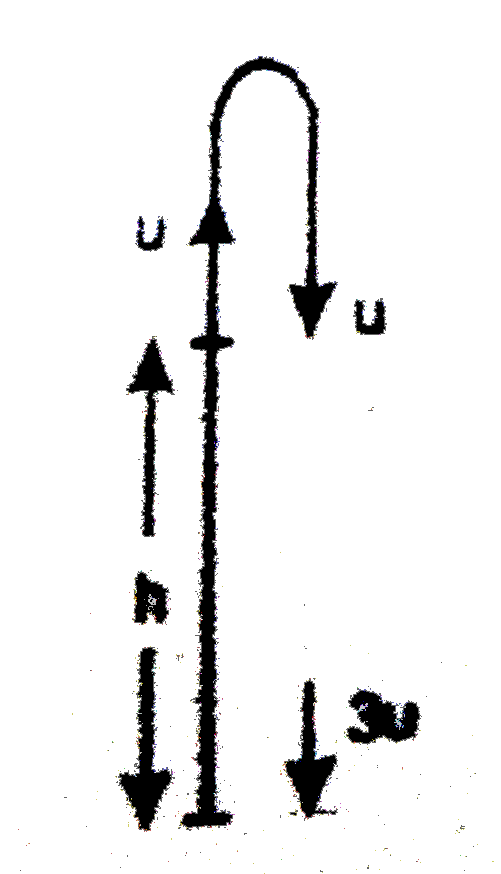# A stone is thrown vertically upwards with an initial speed u from the top of a tower, reaches the ground with a speed 3 u. The height of the tower

139 views
in Physics
closed
A stone is thrown vertically upwards with an initial speed u from the top of a tower, reaches the ground with a speed 3 u. The height of the tower is :
A. (3u^(2))/g
B. (4u^(2))/g
C. (6u^(2))/g
D. (9u^(2))/g

by (84.1k points)
selected1 methode -Let downward direction is taken as +ve. Initial is -ve=u(say)
:. From the equation, v^(2)-u^(2)=as we get (3u)^(2)-(-u)^(2)=20 hg
rArr h=(u^(2))/g "B"
The stone is thrown vertically upward with an initial velocity u from the top a tower it reaches the highest point and returns back and reaches the tope of tower with the same velocity u vertically downwards. Now, from the equation
rArr (3u)^(2)=u^(2)+2gh rArr 2gh =9u^(2)-u^(2) rArr h=(8u^(2))/(2g) rArr h=(4u^(2))/g "B"